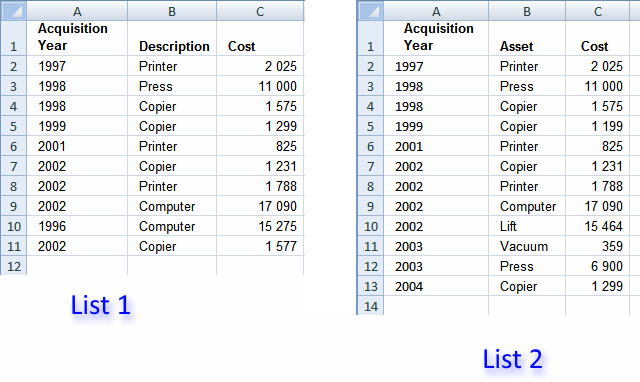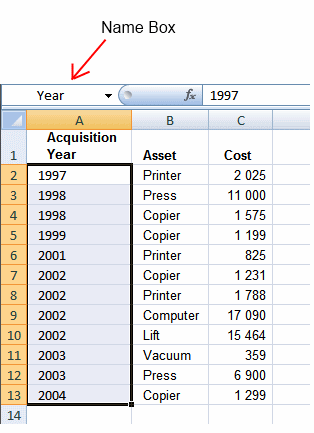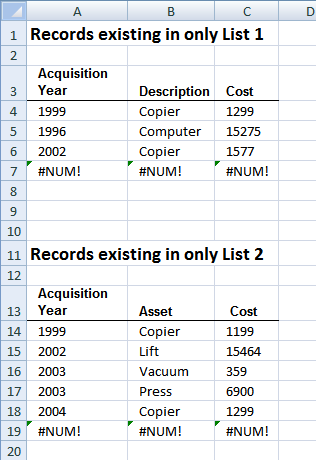Author: Oscar Cronquist Article last updated on January 09, 2019In this example we are going to use two lists with identical columns, shown in the image above. It is easy to modify the COUNTIFS function if your columns are not arranged in the same order, see the COUNTIFS explanation below.

### Create named ranges

There is really no need for named ranges in this example, although they shorten array formulas considerably. You can skip this step if you want.

1. Select A2:A13 on sheet "List 2"
2. Type Year in Name Box
3. Press EnterRepeat with remaining ranges:
Sheet: List 2 , Range:B2:B13, Name: Asset
Sheet: List 2 , Range:C2:C13, Name: Cost
Sheet: List 1 , Range:A2:A11, Name: Year1
Sheet: List 1 , Range:B2:B11, Name: Asset1
Sheet: List 1 , Range:C2:C11, Name: Cost1

### Filter records existing in only one listArray formula in cell A4:

=INDEX('List 1'!\$A\$2:\$C\$11, SMALL(IF(COUNTIFS(Year,  Year1,  Asset,  Asset1,  Cost,  Cost1)=0, ROW(Year1)-MIN(ROW(Year1))+1), ROW(A1)), COLUMN(A1))

To enter an array formula, type the formula in a cell then press and hold CTRL + SHIFT simultaneously, now press Enter once. Release all keys.

The formula bar now shows the formula with a beginning and ending curly bracket telling you that you entered the formula successfully. Don't enter the curly brackets yourself.

Copy cell A4 and paste it to the right as far as needed. Copy cells and paste down as far as needed.

Array formula in cell A14:

=INDEX('List 2'!\$A\$2:\$C\$13, SMALL(IF(COUNTIFS(Year1,  Year,  Asset1,  Asset,  Cost1,  Cost)=0, ROW(Year)-MIN(ROW(Year))+1), ROW(A1)), COLUMN(A1))

Copy cell A4 and paste it to the right as far as needed. Copy cells and paste down as far as needed.

### Explaining the array formula in cell A4

Step 1 - Find records existing only in List 1

Let us take a look at the bolded part of the formula.

=INDEX('List 1'!\$A\$2:\$C\$11, SMALL(IF(COUNTIFS(Year,  Year1,  Asset,  Asset1,  Cost,  Cost1)=0, ROW(Year1)-MIN(ROW(Year1))+1), ROW(A1)), COLUMN(A1))

COUNTIFS(criteria_range1,criteria1, criteria_range2, criteria2...)
The COUNTIFS function counts the number of cells specified by a given set of conditions or criteria

COUNTIFS(Year,  Year1,  Asset,  Asset1,  Cost,  Cost1)

becomes

COUNTIFS({1997, 1998, 1998, 1999, 2001, 2002, 2002, 2002, 2002, 2003, 2003, 2004},{1997, 1998, 1998, 1999, 2001, 2002, 2002, 2002, 1996, 2002}, {Printer, Press, Copier, Copier, Printer, Copier, Printer, Computer, Lift, Vacuum, Press, Copier},{Printer, Press, Copier, Copier, Printer, Copier, Printer, Computer, Computer, Copier},{2025, 11000, 1575, 1199, 825, 1231, 1788, 17090, 15464, 359, 6900, 799},{2025, 11000, 1575, 1299, 825, 1231, 1788, 17090, 15275, 1577})

and returns this array: {1, 1, 1, 0, 1, 1, 1, 1, 0, 0}

COUNTIFS(Year,  Year1,  Asset,  Asset1,  Cost,  Cost1)=0

becomes

{1, 1, 1, 0, 1, 1, 1, 1, 0, 0}=0

and returns

{FALSE, FALSE, FALSE, TRUE, FALSE, FALSE, FALSE, FALSE, TRUE, TRUE}

Step 2 - Convert boolean array to row numbers

The IF function has three arguments, the first one must be a logical expression. If the expression evaluates to TRUE then one thing happens (argument 2) and if FALSE another thing happens (argument 3).

IF(COUNTIFS(Year,  Year1,  Asset,  Asset1,  Cost,  Cost1)=0, ROW(Year1)-MIN(ROW(Year1))+1)

becomes

IF({FALSE, FALSE, FALSE, TRUE, FALSE, FALSE, FALSE, FALSE, TRUE, TRUE}, ROW(Year1)-MIN(ROW(Year1))+1)

becomes

IF({FALSE, FALSE, FALSE, TRUE, FALSE, FALSE, FALSE, FALSE, TRUE, TRUE}, {1, 2, 3, 4, 5, 6, 7, 8, 9, 10})

and returns

{FALSE, FALSE, FALSE, 4, FALSE, FALSE, FALSE, FALSE, 9, 10}

Step 3 - Return the k-th smallest value

To be able to return a new value in a cell each I use the SMALL function to filter column numbers from smallest to largest.

The ROW function keeps track of the numbers based on a relative cell reference. It will change as the formula is copied to the cells below.

SMALL(IF(COUNTIFS(Year,  Year1,  Asset,  Asset1,  Cost,  Cost1)=0, ROW(Year1)-MIN(ROW(Year1))+1), ROW(A1))

becomes

SMALL({FALSE, FALSE, FALSE, 4, FALSE, FALSE, FALSE, FALSE, 9, 10}, ROW(A1))

becomes

SMALL({FALSE, FALSE, FALSE, 4, FALSE, FALSE, FALSE, FALSE, 9, 10}, 1)

and returns 4.

Step 4 - Return a value of the cell at the intersection of a particular row and column

The INDEX function returns a value based on a cell reference and column/row numbers.

INDEX('List 1'!\$A\$2:\$C\$11, SMALL(IF(COUNTIFS(Year,  Year1,  Asset,  Asset1,  Cost,  Cost1)=0, ROW(Year1)-MIN(ROW(Year1))+1), ROW(A1)), COLUMN(A1))

becomes

=INDEX('List 1'!\$A\$2:\$C\$11, 4, COLUMN(A1))

becomes

=INDEX('List 1'!\$A\$2:\$C\$11, 4, 1)

and returns 1999.